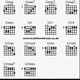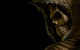# 3D graphing

A 3D version of the calculator would be great - we don't have that feature built in yet, but hopefully someday soon!

In the meantime, you can mimic 3D graphing like this: https://www.desmos.com/calculator/nqom2ih05g

31 out of 31 found this helpful
Have more questions? Submit a request

•Darby Rathbone

you should also have a point that changes the view of the 3d graph kindof like this one https://www.desmos.com/calculator/shw1wthey5

•Mike Riberdy

Is this a project or just a subjunctive situation?

•Dakotais

How can I graph my own things on this? The formulas are so complex, I don't know where to put my functions!

•Ansgar F26

You should also consider to have a perspective, zooming and create the possibility of rotating the graph with a point and with sliders so that you can also animate a axis. Made the whole chart also a bit more clear and added some more explanatory comments.

•Ryan Eastman-morris

wtf jkjk

•Joseph A

Has anyone here played Eye Wire before? If not, then go check it out. It solves the problem of 3D movement by allowing you to scroll through a cross-section of the block. The same could be applied to graphs with 3 variables.

BTW, Eye Wire is a cool game ;-)

•2165404

A bit confusing.

•Informationaether

With something like this: https://www.desmos.com/calculator/omcaw59kxc

You can even plot the solution to an equation f(x, y, z) = 0

Sorry its extremely laggy - turns out doing this requires a lot of calculating.

•Saeid Nourian

Desmos is great for 2D graphs, but 3D plotting is computation-intense and require GPU processing.
For real-time rendering of 3D equations you can try this:
http://www.runiter.com/graphing-calculator/

•Per H. Christiansen
•Per H. Christiansen
•eGamer Me

This is an enhanced version from the one made by Ansgar F26. It can accept any function now, instead of being stuck to only using f, g and h.

https://www.desmos.com/calculator/lqe0zjjgzc

•Per H. Christiansen

Would someone be kind enough to help me by showing (if possible) how to place the point of intersection (s1, s2, s3) between the plane and the line through the points (i) and (r), so that it lies on the line and not next to it. The coordinates seem to be calculated correctly, but the placement in the coordinate system turns out wrong:

Line-plane-intersection

Edited by Per H. Christiansen
•Per H. Christiansen

Well, after redrawing the line the point of intersection seems to be right on spot:

Line-plane-intersection

Edited by Per H. Christiansen
•Per H. Christiansen

Intersection of two lines in 3d:

Line-line-intersection

•Per H. Christiansen
Edited by Per H. Christiansen
•Joe Quinn

@DarbyRathbone That is an amazing tool, thanks so much! The thing that rules about it is that I can replace your function with what I want and all the stuff you designed still works on it.

Also @SaeidNourian thanks for that link! As cool as the workaround is, it's nice to not need a workaround.

•Andrew Osborne

Here's an example of a 3D graph with perspective: https://www.desmos.com/calculator/rwcweuncgp

Here's an example of what can be done with such a thing: https://www.desmos.com/calculator/kd0cyidgtx

Here's how one may go about creating one: https://www.desmos.com/calculator/m6kzuslsr6

Here's one that interprets 3D functions like the ones in Informationaether's graph, but with perspective: https://www.desmos.com/calculator/wwwwtli3ss

Here's an example of what can be done with the function method: https://www.desmos.com/calculator/s77y1vtzsb

•Xavier Wehrmeister

https://www.desmos.com/calculator/razfq8pjev
I took the 3D graph and added moveable points w/ labels to make rotating the graph more intuitive. =)

•Pritt Balagopal

The parametric 3D graph is good, but it doesn't allow graphing of explicit relations though. Only equations of the form z=f(x,y) can be graphed.

•Owen J

It dosn't have to be 3D it just has to look 3D. Like this.

•1s2s2p

I know I'm a bit late this this, but I've worked on this: https://www.desmos.com/calculator/d8r6p3erot, quite simple. It doesn't show the 3D graph, but does show the x and y slices for a point on a multivariable graph.

Setting a=2 will show what the graph looks like when y is kept constant at 2, and shows the change in x. Meanwhile, setting b=-5, shows what the path of y looks like when x is kept constant at -5. red axis is for y, and green axis is for x.

This can be shown here by setting z=f(x,y), x=b and y=a

https://www.geogebra.org/3d?lang=en

Edited by 1s2s2p
•Mniehaus19

Yah I don't get any of this.

•Instinctify

Here's a 3D surface analyzer I wrote for use with Osserman's 'Two-Dimensional Calculus'

https://www.desmos.com/calculator/mofxxgq4ag

I kept it simple (19 lines of code) for easy re-purposing

Edited by Instinctify